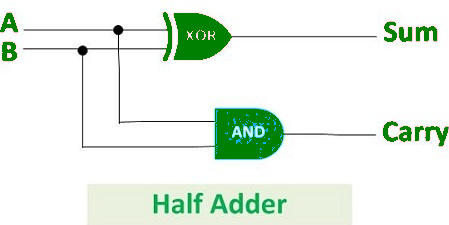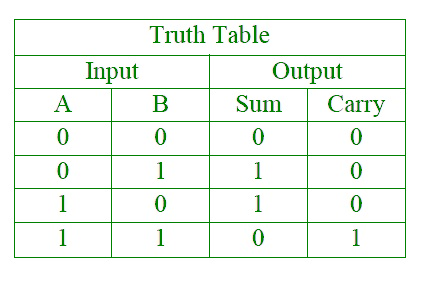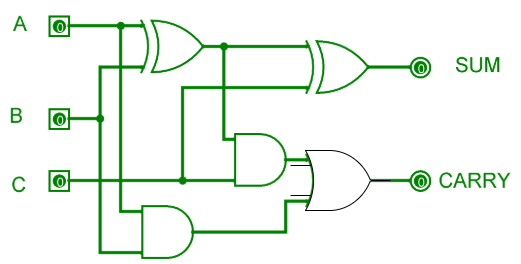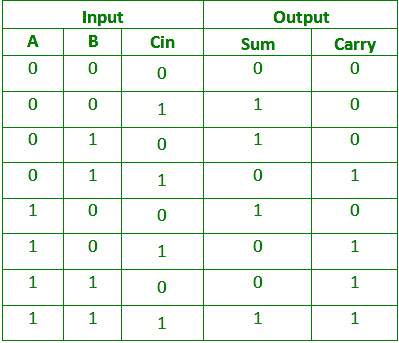Half Adder is a combinational logic circuit which is designed by connecting one EX-OR gate and one AND gate. The half adder circuit has two inputs: A and B, which add two input digits and generates a carry and a sum.The output obtained from the EX-OR gate is the sum of the two numbers while that obtained by AND gate is the carry. There will be no forwarding of carry addition because there is no logic gate to process that. Thus, this is called Half Adder circuit.

Logical Expression :

```Sum = A XOR B
Carry = A AND B
```

Truth Table :Full Adder is the circuit which consists of two EX-OR gates, two AND gates and one OR gate. Full Adder is the adder which adds three inputs and produces two outputs which consists of two EX-OR gates, two AND gates and one OR gate. The first two inputs are A and B and the third input is an input carry as C-IN. The output carry is designated as C-OUT and the normal output is designated as S which is SUM.Equation obtained by EX-OR gate is the sum of the binary digits. While the output obtained by AND gate is the carry obtained by addition.

Truth Table :Logical Expression :

```SUM = (A XOR B) XOR Cin = (A ⊕ B) ⊕ Cin
CARRY-OUT = A AND B OR Cin(A XOR B) = A.B + Cin(A ⊕ B)
```

1 Half Adder is combinational logic circuit which adds two 1-bit digits. The half adder produces a sum of the two inputs. Full adder is combinational logical circuit that performs an addition operation on three one-bit binary numbers. The full adder produces a sum of the three inputs and carry value.
2 Previous carry is not used. Previous carry is used.
3 In Half adder there are two input bits ( A, B). In full adder there are three input bits (A, B, C-in).
4 Logical Expression for half adder is :
S=a⊕b ; C=a*b.
Logical Expression for Full adder is :
S=a⊕b⊕Cin; Cout=(a*b)+(Cin*(a⊕b)).
5 It consists of one EX-OR gate and one AND gate. It consists of two EX-OR, two AND gate and one OR gate.
6 It is used in Calculators, computers, digital measuring devices etc. It is used in Multiple bit addition, digital processors etc.

Attention reader! Don’t stop learning now. Get hold of all the important CS Theory concepts for SDE interviews with the CS Theory Course at a student-friendly price and become industry ready.

My Personal Notes arrow_drop_upIf you like GeeksforGeeks and would like to contribute, you can also write an article using contribute.geeksforgeeks.org or mail your article to contribute@geeksforgeeks.org. See your article appearing on the GeeksforGeeks main page and help other Geeks.

Please Improve this article if you find anything incorrect by clicking on the "Improve Article" button below.

Improved By : krishshewani7

2

Please write to us at contribute@geeksforgeeks.org to report any issue with the above content.